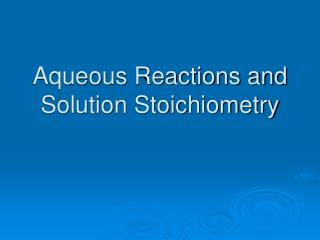DownloadDownload PresentationAqueous Reactions and Solution Stoichiometry

# Aqueous Reactions and Solution Stoichiometry

Télécharger la présentation## Aqueous Reactions and Solution Stoichiometry

- - - - - - - - - - - - - - - - - - - - - - - - - - - E N D - - - - - - - - - - - - - - - - - - - - - - - - - - -
##### Presentation Transcript

1. Aqueous Reactions and Solution Stoichiometry

2. Know the symbols • g • s • l • aq • c

3. What is the difference between a “liquid solution” and an “aqueous solution” ?

4. Which of the following would be an electrolytic or non-electrolytic solution ? • NO • NaCl • PCl3 • CO2 • MgSO4

5. Weak vs Strong Electrolytes • Use a single arrow to show a reaction with a strong electrolyte. • Use a double arrow to show a reaction with a weak electrolyte.

6. Precipitation Reactions • Precipitation reactions are reactions that result in the formation of an insoluble product. • Make sure to use an “s” to designate the solid that precipitates.

7. Solubility Rules • You must memorize the solubility rules for the AP exam and for a quiz in class.

8. Ionic Equations • You need to know the difference between the following types of equations: • Molecular equations • Complete ion equations • Net ionic equations • What is a spectator ion?

9. How to write a “Net Ionic Equation” • Write a balanced molecular equation. • Rewrite the equation showing all strong electrolytes that dissociate (or ionize) in solution. • Cancel out spectator ions.

10. Acid Base Reactions • You need to memorize the list of strong acids and bases. • Acids: • HCl • HBr • HI • HClO3 chloric acid • HClO4 perchloric acid • HNO3 nitric acid • H2SO4 sulfuric acid

11. Bases: • Group 1A metal hydroxides (LiOH, NaOH, KOH, RbOH, CsOH) • Heavy group 2A metal hydroxides • Ca(OH)2 , Sr(OH)2 , Ba(OH)2

12. What do you get when you mix an acid and a base? • Any thoughts? • A neutralization reaction • Can you explain how the water is produced in a neutralization reaction?

13. Oxidation-Reduction Reactions • OIL oxidation is loss of electrons • RIG reduction is gain of electrons • See the textbook for the rules for assigning oxidation numbers.

14. Activity Series • You can use the activity series to determine if some reactions involving metals will occur. • An activity series is a list of metals arranged in order of decreasing ease of oxidation. • See the textbook for the activity series diagram.

15. You will need to memorize the activity series for the AP exam. • Look at the AP Reference packet. Take a good look at the Standard Reduction Potential chart.

16. Molarity moles of solute Molarity = liters of solution Molarity is another way to express concentration.

17. Molality moles of solute Molality = kilograms of solvent Molality is another way to express concentration.

18. Dilution MiVi = MfVf i is the initial concentration f is the final concentration

19. Titrations • Use titrations to determine the concentration of a solution. • The point in a titration when stoichiometrically amounts are brought together is called the equivalence point. • You titrate an unknown solution with a standard solution. Use an indicator to show when the equivalence point has been reached. • The color change during a titration signals the endpoint which is very close to the equivalence point.

20. Titration Calculations • If you are given a volume and molarity for a substance, start the calculation with that. • Convert to moles of what you know • then use the molar ratios to convert to moles of the unknown substance • Finally, use the volume of the unknown substance to convert to molarity.

21. Titrations • You can use the dilution equation to solve titration problems, as long as the molar ratio is 1 to 1.

22. Problems to Try • 5, 6, 7, 9, 10, 11, 13, 16, 17, 18, 21, 26, 27, 28, 35, 37, 39, 41, 43, 46, 51, 53, 54, 56, 59, 60, 63, 65, 66, 67, 69, 71, 73, 76, 78, 81, 89, 90